# Translations of Encyclopedia about Mathematics

## Counting with Decimal Numbers

When counting with decimal numbers, the same rules apply as when counting with other numbers. The important point is that the decimal point remain in the proper position. Some examples:

3.25 + 6.54 = 9.79

or

 18.45 DM + 20.65 DM 39.10 DM

6.54 – 3.25 = 3.29

or

 20.65 DM - 18.45 DM 2.20 DM

4.53 . 2.1 = 9.513

or

 4.53 * 2.1 = 9.513 9 06 0 45 3 9 51 3

The number of positions after the decimal point of individual multipliers are added together (in our case a total of three).

12.8148 : 5.43 = 2.36

or

 12.8148 : 5.43 = 2.36 (before starting, multiply both numbers by a factor or ten until there is no longer any decimal point) 128148 : 54300 = 2.36 108600 0195480 0162900 00325800 00325800 00000000

Translating Spanish Portuguese Translations Portuguese Spanish Translating Hungarian Dutch Translations Dutch Hungarian Translating Polish

## Counting with Fractions

Fractions serve to help us count with a part of a whole. The rules for counting with fractions are the same as when adding with other numbers (natural or whole) except for the fact that it is at first difficult to recognise them due to a different method of writing them and their more difficult use.

The basic idea behind adding fractions is the following: if we want to divide a piece of cake into four same-sized portions, we would get four quarters.

1/4 is referred to as one quarter.

Other commonly used fractions are ½, which is one half, or ¾, which is three quarters.

When adding fractions, there are one of three methods we can use, depending on whether we are:

1. adding fractions which have the same denominator: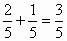In this case, the individual nominators are added together while the denominator remains the same.

2. adding fractions with different denominators: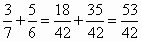Fractions which do not have the same denominator can be added together by first converting them so that they have the same denominators, which is accomplished by expanding the fractions (multiplying each nominator and denominator by the same number) until all the fractions have the same denominator. Once this is accomplished, we can add the nominators while, as before, keeping the denominators the same.

by a) converting all fractions so that all their denominators are the same and adding them together:

b) adding all whole numbers separately and fractions separately once they have been converted so that their denominators are all the same: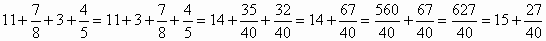Subtracting Fractions

When subtracting fractions, there are three possible procedures we can take, according to whether:

1. we are subtracting fractions with the same denominator: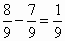In this case, the denominator stays the same and only the nominators are subtracted.

2. we are subtracting fractions with different denominators: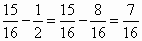In this case, the subtraction is only possible after converting all the fractions so that they have the same denominator, after which the denominators remain the same and only the nominators are subtracted.

3. when subtracting mixed numbers, we can proceed either by:
1. converting all the numbers into fractions with the same denominator and then subtracting as previously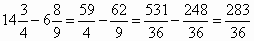2. or by first calculating the whole numbers and then the fractions, after converting them all so that they have the same denominator, keeping the denominator the same and only subtracting the nominators. Otherwise, some of the whole numbers may be converted to fractions with the same denominator, if necessary.Multiplying Fractions

Fractions can be multiplied by simply multiplying their denominators and nominators against each other. Mixed or whole numbers are first converted into fractions.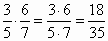Some fractions can be simplified, making the overall equation easier to compute: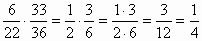In this case, the value 33 was shortened against 22 (to give a fraction of 3/2) and 6 against 36 (to give a fraction of 1/6). After that, the denominators and nominators are multiplied. As we can see, our previous shortening made our task much easier.

Dividing Fractions

A fraction can be divided by another fraction by simply reversing the denominator and nominator of the fraction we are dividing and multiplying rather than dividing.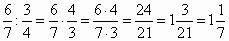The rules which apply when calculating with brackets and whole or real numbers also apply with brackets and fractions.

Translating Russian Ukrainian Translations Ukrainian Russian Translating Persian Arab Translations Arab Persian Translating TurkishEnter your search terms Submit search form